Courses

# Test: Units & Measurements

## 25 Questions MCQ Test Physics For JEE | Test: Units & Measurements

Description
This mock test of Test: Units & Measurements for Class 11 helps you for every Class 11 entrance exam. This contains 25 Multiple Choice Questions for Class 11 Test: Units & Measurements (mcq) to study with solutions a complete question bank. The solved questions answers in this Test: Units & Measurements quiz give you a good mix of easy questions and tough questions. Class 11 students definitely take this Test: Units & Measurements exercise for a better result in the exam. You can find other Test: Units & Measurements extra questions, long questions & short questions for Class 11 on EduRev as well by searching above.
QUESTION: 1

### Which of the following sets cannot enter into the list of fundamental quantities in any system of units ?

Solution:

We define length and time separately as it is not possible to define velocity without using these quantities. This means that one fundamental quantity depends on the other. So, these quantities cannot be listed as fundamental quantities in any system of units.

QUESTION: 2

### Which of the following is not the unit of time

Solution:

Parallactic second is the unit of distance because parallactic second is an abbreviation of parsec. Parsec = Parsec is the Unit for larger distances.It is the distance at which a star would make parallax of one Second of arc.

QUESTION: 3

### The unit of impulse is the same as that of :

Solution:

The newton second (also newton-second, symbol N s or N.s) is the derived SI unit of impulse. It is dimensionally equivalent to the momentum unit kilogram metre per second (kg.m/s). One newton second corresponds to a one-newton force applied for one second.

QUESTION: 4

Which of the following is not the unit of energy?

Solution:

F = ma
[F] = kg m/s2

QUESTION: 5

If the unit of length is micrometer and the unit of time is microsecond, the unit of velocity will be :

Solution:

Unit of velocity = m/s
1m = 106  Micro meter
And 1 sec = 106  Micro sec
Thus 1 m/s = 106 106  micro meter /micro second

QUESTION: 6

What is the physical quantity whose dimensions are M L2 T-2 ?

Solution:

Dimension of KE is ML2T-2
That of pressure is ML-2T-2
That of momentum is ML/T
That of power is ML2T-3

QUESTION: 7

A unitless quantity :

Solution:

A dimensionless quantity can have unit. for example angle (radian). But oppisite is not true. A unitless quantity can never have dimensions.it is the unit that give dimensions.

QUESTION: 8

If a and b are two physical quantities having different dimensions then which of the following can denote a new physical quantity

Solution:

Quantities with different dimensions cant be added or subtracted. Also the dimension of the product of the quantities in power is always one. But when two quantities are multiplied the dimension of the product is the product of the dimensions of the initial quantities.

QUESTION: 9

Two physical quantities whose dimensions are not same, cannot be :

Solution:

The two physical quantities which do not have the same dimensions can’t be added or subtracted in the same expression because doing so would lead to equating two quantities of different dimensions, which is non possible. Ex [F]  [P].

QUESTION: 10

Choose the correct statement (S)

Solution:

Explanation : a) A topological sort of a directed acyclic graph (DAG) is any ordering m1, m2, …, mn of the nodes

of the graph, such that if mimj is an edge then mi appears before mj . Any topological sort of a

the dependency graph gives a valid evaluation order for the semantic rules.

b) The parse tree can be annotated with synthesized or inherited attributes. The parse tree can also be indicated with an arrow mark to indicate the manner in which the value gets propagated between the nodes of the parse tree. This graph is called as dependency graph as it indicates the dependency between nodes for deriving the values. This graph is an acyclic graph which doesn’t have a cycle. The presence of a cycle indicates that the graph is incorrect as the dependence of nodes for deriving values cannot be predicted. Edges in the dependence graph show the evaluation order for attribute values and thus the graph is a directed one.

QUESTION: 11

Planck's constant has the dimensions of :

Solution:

We know that E = hv , where E is energy and v is frequency.
Thus we get h = E/v
And [h] = [E/v] = ML2T-2 / T-1
= ML2T-1
[P] = MLT-1
[F] = MLT-2
[Angular momentum] = [P x r] = ML2T-1

QUESTION: 12

Electron volt is a unit of

Solution:
QUESTION: 13

The dimensional formula of RT is same as that of:

Solution:

The vander Waals gas equation is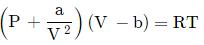where P is the pressure, V is molar volume and T is the temperature of the given sample of gas. R is called mola gas constant, a and b are called vander Waals constants.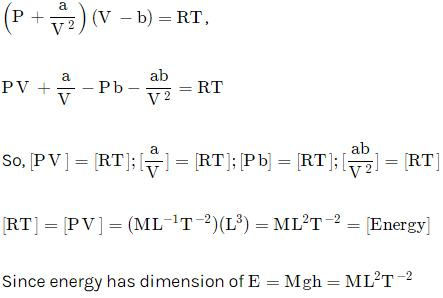QUESTION: 14

Which pair of following quantities has dimensions different from each other

Solution:

Moment of inertia =1/2​×mass×(Radius of gyration)2=[M1L2T0]
Moment of force = torque =N.m=[M1L2T−2]
Both are different from each other.

QUESTION: 15

If the error in measurement of radius of sphere is 1% , what will be the error in measurement of volume

Solution:

Volume=4πR3/3
ΔV/V×100=3∆R/R×100
=3×1/100​×100
=3
∴ Percentage error in volume is 3%

QUESTION: 16

The velocity 'v' (in cm/s) of a particle is given in terms of time 't' (in s) by the equation

v = at +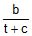The dimensions of a, b and c are

Solution:

As v = at + b / (t + c)
We get that v, at and b / (t+c) have the same dimensions as they are equated and added.
Similarly c and t have also same dimensions, [c] = T and [a] = L/T
And [b] = L

QUESTION: 17

In case of measurement of ‘g’, if error in measurement of length of pendulum is 2%, the percentage error in time period is1 %. The maximum error in measurement of g is

Solution:

Time period of oscillation of pendulam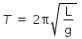where L is length of pendulam and g is acceleration due to gravity.
hence we have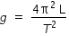...........................(1)
hence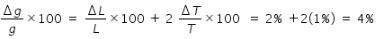QUESTION: 18

The time dependence of a physical quantity ?

P = P0exp(_at2)

where a is a constant and t is time. The constant a

Solution:

We know that any quantity in power as a dimension of 1, thus [at2] = 1
Thus we get [a] = T-2

QUESTION: 19

Force F is given in terms of time t and distance x by

F = A sin C t + B cos D x

Then the dimensions of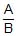and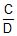are given by

Solution:

Dimension of [A]=[MLT-2]
Dimension of [B]=[MLT-2]

[A]/[B]= [ M0L0T0]
CT=1
Dimension of  [C]=1/[T]=[T-1]
DL=1
Dimension of  [D]=1/[L]=[L-1]
[C][D] =[T-1]/[L-1]
=LT-1

A/B=Force/Force=[M0L0T0]
Ct=∠⇒C=Angle/Time=1/T=T-1
Dx=∠⇒D=Angle/Distance=1/L=L-1
∴C/D=T-1L-1 =[M0 LT-1 ]

QUESTION: 20

The Van der Waal equation for 1 mole of a real gas is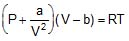where P is the pressure, V is the volume, T is the absolute temperature, R is the molar gas constant and a, b are Van dar Waal constants. The dimensions of a are the same as those of

Solution:

Solution,
As we know the Vander Waals equation is {P+(a/V2)} (V−b) =RT
Then,
The dimension of a is,
(a/V2) = (P)
Or, a=PV2

QUESTION: 21

The dimensional formula of coefficient of viscosity is

Solution:
QUESTION: 22

If force (F) is given by F = Pt_1 + Qt, where t is time. The unit of P is same as that of

Solution:

According to the principle of homogeneity of dimension, an equation is dimensionally correct when each term has same dimension on both sides of the equation.

Since left side has dimension of force so the term Pt−1 will have also dimension of force.

Thus, [P][T−1]=[F]=[MLT−2]

or [P]=[MLT−1]

We know that the dimension of momentum is [p]=[MLT−1]

QUESTION: 23

The product of energy and time is called action. The dimensional formula for action is same as that for

Solution:

Both are different from each other.
Energy × Time=(M1L2T−2)×(T1)=M1L2T−1
Force × Velocity=(M1L1T−2)×(L1T−1)=M1L2T−3
Impulse × distance=(M1L1T−1)×(L1)=M1L2T−1
Power=M1L2T−3
Angular Energy=M1L1T−2

QUESTION: 24

When a wave traverses a medium, the displacement of a particle located at x at time t is given by y = a sin (bt - cx) where a, b and c are constants of the wave. The dimensions of b/c are the same as those of

Solution:

Argument of sin is dimensionless and using principle of dimensional homogeneity, we get
∣bt∣=∣cx∣=[M0L0T0]
Thus, ∣b∣=T−1 and ∣c∣=[L−1]
Thus, dimensions of b/c are [LT−1], which is the same as the velocity of wave.

QUESTION: 25

In the above question dimensions of b/c are the same as those of

Solution: